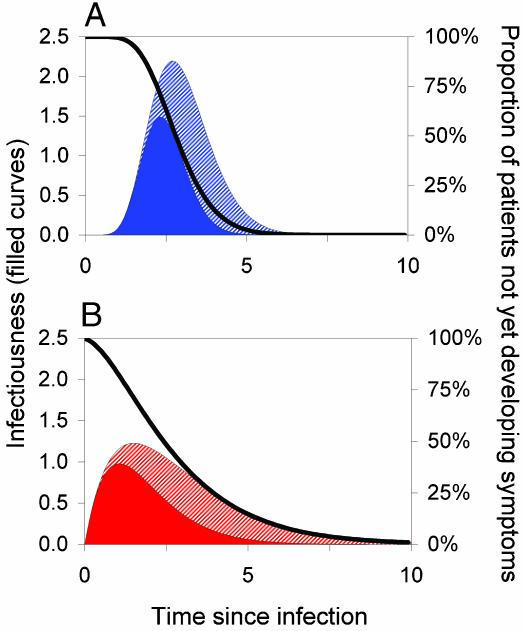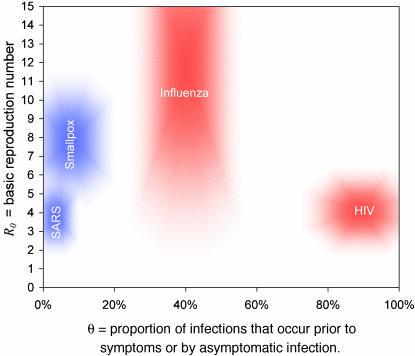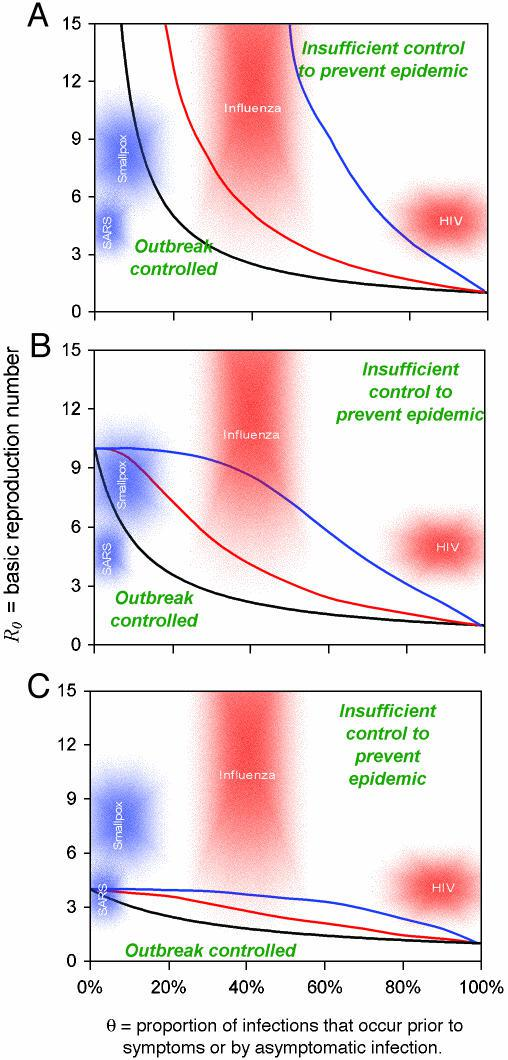# SARI数据分析(2): 使传染病疫情可以控制的因素

Factors that make an infectious disease outbreak controllable

### 传染病爆发的模拟¶• 描述为从感染后时间的函数（以任意单位测量）。
• 实线表示随时间推移的传染性（左轴）。黑色曲线表示S(τ)，即人在一定时间（右轴）内未出现症状的概率。
• 基本再生数 R0 为传染性曲线下面积（实心颜色加斜线部分）。
• 实心区域代表症状之前出现的传播，因此 θ定义为症状前传播的比例，是实色的传染性曲线下面积与总面积的比例。
• （A 和 B）显示潜伏期和传染性的不同分布。两种情况下均具有 R0 = 5、Tg = 3（以任意时间单位）和 θ = 0.5；A 显示低方差 $0.1 \times mean^2$ ,B 显示高方差$0.5 \times mean^2$

• 基本再生数(basic reproduction number)，R0，定义为易感人群中由初次感染引起的二次感染的数量，从而测量病原体的内在传播能力; 它可以计算为传染性曲线下的面积(见图1和公式3). 一个流行病要在传播的早期阶段扩散，必须有不止一个次要病例由主要病例产生，因此我们需要 R0>1。
• 疾病发生时间(disease generation time) Tg, 是指个体感染者与感染者之间的平均时间间隔「我理解是一个人传染上疾病到他传染下一个人之间的时间」，它与 R0一起确定了疫情发展的时间尺度，从而确定了采取干预措施避免大规模疫情爆发的速度。 具体地说，在一个不断增长的暴发中，病例数的倍增时间为$ln (2) Tg/(R0 - 1)$「此处有歧义, 原文中ln(2)中的2为指向文献的超链接, 不确定是否是数字
• 发生出现症状之前(或无症状)的传染比例θ ，这决定了基于症状的公共卫生控制措施减少感染数量的潜力。### 数学模型的建立和分析¶

The basic model of disease transmission determines the dynamics of Y (t,τ), the current number of people, at time t, who were infected time c ago. The cumulative epidemic size by time t is given by Y(t) = ∫∞0 Y(t, τ) dτ, whereas the incidence of infection (i.e., rate of people acquiring infection) at time t is Λ(t) = Y(t, 0). The model predicts that an outbreak will be controlled if the incidence declines to zero, i.e., Λ(t) → 0 as t becomes large. The model of disease spread is determined by the Von Foerster equations (15),

Y(t,τ): 在t时刻, 当前被感染的人数, 这些人是在τ时间之前被感染的.

$$\frac{\partial Y(t, \tau)}{\partial t}+\frac{\partial Y(t, \tau)}{\partial \tau}=0 \tag 1$$$$Y(t, 0)=\int_{0}^{t} \beta(\tau) Y(t, \tau) d \tau \tag 2$$

$$R_0=\int_{0}^{\infty} \beta(\tau) d \tau \tag 3$$

$$T_g=\frac{\int_{0}^{\infty} \tau \beta(\tau) d \tau}{\int_{0}^{\infty} \beta(\tau) d \tau} \tag 4$$

$$Y(t, 0)=\int_{0}^{t} \beta(\tau) [1-ε_I+ε_I S(\tau)] Y(t, \tau) d \tau \tag 5$$
In [ ]:


In [ ]:


In [ ]:



In [ ]: